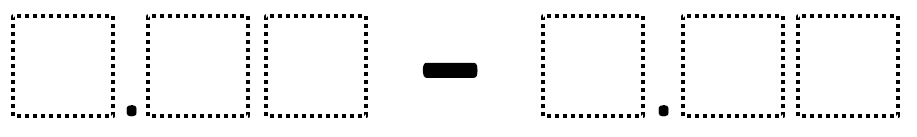# Subtracting Decimals (Elementary)

Directions: Using the digits 1 to 9 at most one time each, fill in the boxes to make the smallest (or largest) difference.### Hint

What number does each box represent?

No answers yet.  If you have one, post it in the comments.

Source: Robert Kaplinsky

## Equivalent Expressions with Fractions

Directions: Using the digits 0 to 9 at most one time each and choosing either …

1.My fourth grade students came up with 0.14 is the smallest difference. This could be achieved by a few different ways. However, the tenths and hundredths had to be ___.12 – ___.98

•That’s what we got too!

•But you can’t use 0s, you can only use whole number digits 1-9.

•the awnser is 1.23-2.45

2.We thought about negative numbers. My class came up with -9.36 if you consider negative numbers.

•I’m rethinking our logic now. The smallest difference would mean show two numbers that are as close as possible on a number line.

3.My fifth graders also got .14 as the smallest difference, but we did it using the digits 1-9. Both 4.12-3.98 and 5.12-4.98 can give you this result.

They also figured 9.87-1.23 = 8.64 as the largest difference.

4.My 4/5th grade students also got 0.14 several ways using _.12-_.98= 0.14. We did not use negative numbers.

5.1.3 -0.42 =.98

6.wroung on 2.9-1.8=1.1